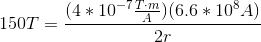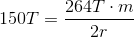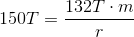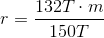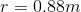# High School Physics : Understanding Magnetic Fields

## Example Questions

### Example Question #61 : Electricity And Magnetism

A current of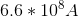runs through a straight wire. If the resulting magnetic field is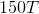, what is the radius of the field?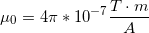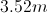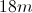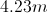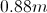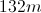Explanation:

Ampere's law states: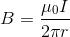.

In other words, the magnetic field (), is equal to a constant (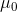) times the current (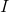) divided by the circumference of the magnetic field it is creating.

We are given the current, the constant, and the magnetic field strength. Using these values, we can solve for the magnetic field radius.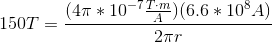Notice that thecancels out.